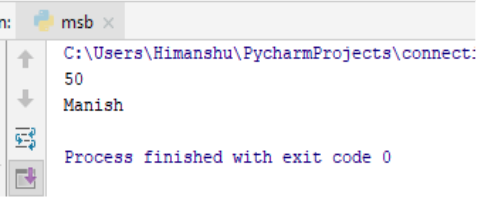×

Html & Html5

Introduction to Html

Component of Html

Html Form

Html5 basics

Html5 Grid

Html5 Graphics

Html5 Media

Html5 APIs

Python and Pandas

Introduction to Python

Component of Python

Python Variables

Here in Python, there is no special command to create the variables. As soon as you assign a value ,in that very moment your variable is been created.

Let's check it out :-

a=50

b="Manish"

print(a)

print(b)

Output of the Program

So here in Python it’s very easy to create the variable . As in above example , we saw that we were just providing the value to ‘a’ and ‘b’ and than we gave the command of printing these values.
So let’s see...Tips to remember while creating variables

• Variables can start through a single alphabet , like (x,y,z).
• It can start through words , like (age, username).
• It can’t start by numbers.
• It can contain underscore character too , like (Item_kitchen).
• It can contain alpha-numeric characters , like (Manish121).
• It is very case sensitive ,like (Man, MAN,man).

python training insitute| Best IT Training classes in Gurgaon | python training